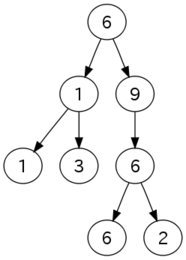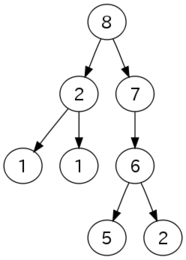시간 제한 메모리 제한 제출 정답 맞은 사람 정답 비율
1 초 256 MB 17 12 8 61.538%

## 문제

You are given a rooted tree. Each vertex of the tree is labeled with an integer. If you pay one dollar, you can change (increment or decrement) the label of a vertex by one.

You want to change the labels such that, for each vertex, its label is strictly greater than any of the labels assigned to its children. Compute the minimum cost required to satisfy this condition.

## 입력

The first line contains two integers: $N$, the number of vertices in the tree, and $C_1$, the label assigned to the root. The vertices are numbered $1$ through $N$, and the root is vertex $1$ ($1 \le N \le 10^5$).

The next $N - 1$ line describe non-root vertices. The $i$-th line contains two integers: $P_i$, the number of the parent of the vertex $i$, and $C_i$, the label assigned to the vertex $i$ ($1 \le P_i < i$, $-10^9 \le c_i \le 10^9$).

## 출력

Print the minimum cost on a single line.

## 예제 입력 1

8 6
1 1
2 1
2 3
1 9
5 6
6 6
6 2


## 예제 출력 1

8


## 예제 입력 2

4 5
1 5
2 5
3 5


## 예제 출력 2

4


## 힌트

The figure on the left is the input configuration for the first sample. The figure on the right is a possible final configuration: the label of each parent is strictly greater than that of its child.